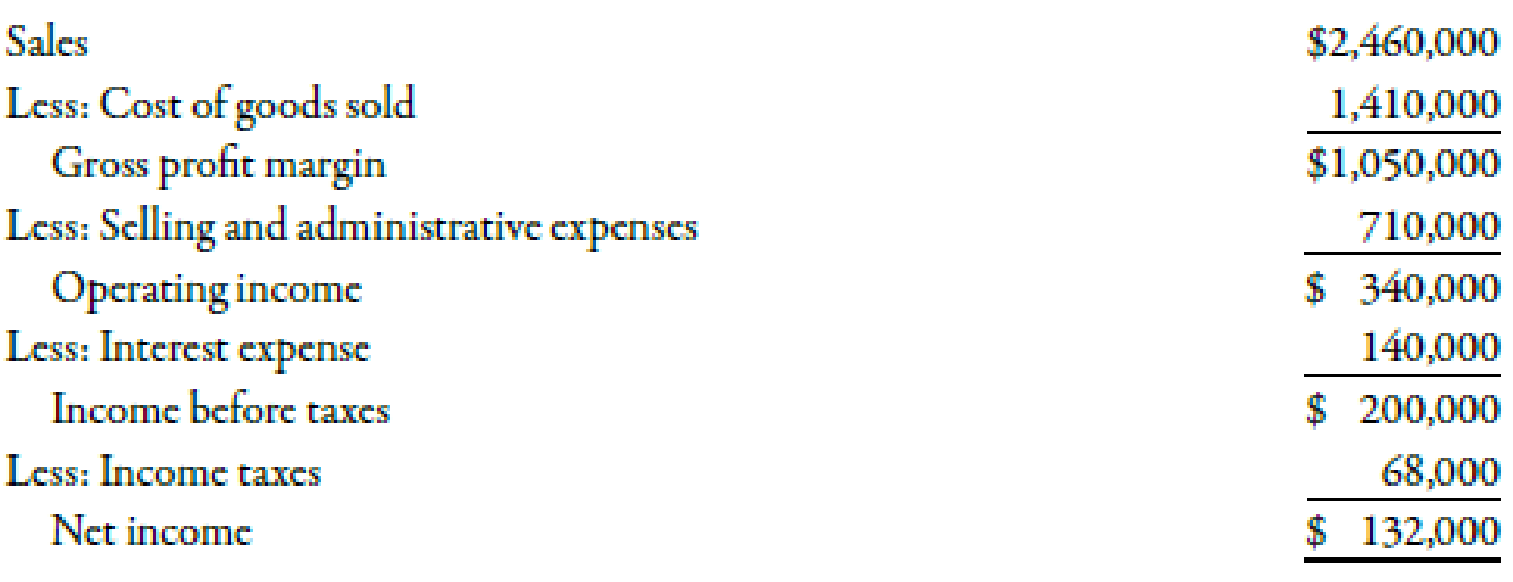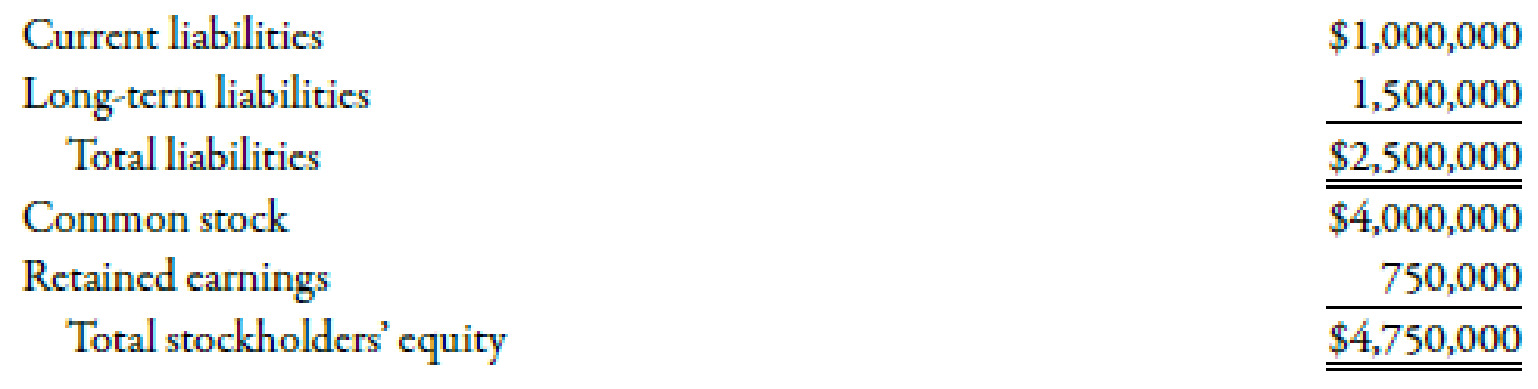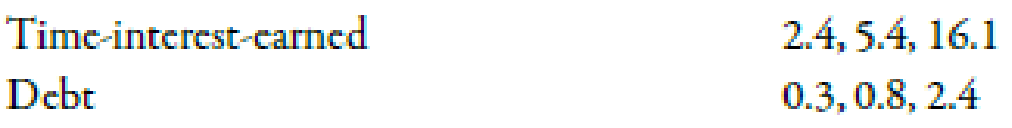# Grammatico Company has just completed its third year of operations. The income statement is as follows: Selected information from the balance sheet is as follows: Required: Note: Round answers to two decimal places. 1. Compute the times-interest-earned ratio. 2. Compute the debt ratio. 3. CONCEPTUAL CONNECTION Assume that the lower quartile, median, and upper quartile values for debt and times-interest-earned ratios in Grammatico’s industry are as follows: How does Grammatico compare with the industrial norms? Does it have too much debt?### Managerial Accounting: The Corners...

7th Edition
Maryanne M. Mowen + 2 others
Publisher: Cengage Learning
ISBN: 9781337115773

#### Solutions

Chapter
Section### Managerial Accounting: The Corners...

7th Edition
Maryanne M. Mowen + 2 others
Publisher: Cengage Learning
ISBN: 9781337115773
Chapter 15, Problem 57P
Textbook Problem
1 views

## Grammatico Company has just completed its third year of operations. The income statement is as follows:Selected information from the balance sheet is as follows:Required:Note: Round answers to two decimal places. 1. Compute the times-interest-earned ratio. 2. Compute the debt ratio. 3. CONCEPTUAL CONNECTION Assume that the lower quartile, median, and upper quartile values for debt and times-interest-earned ratios in Grammatico’s industry are as follows:How does Grammatico compare with the industrial norms? Does it have too much debt?

1.

To determine

Calculate times interest earned ratio.

### Explanation of Solution

Times Interest Earned Ratio

Times interest earned reflects firm’s capability to make provision for its debt. It is computed by adding interest expense before tax to income before tax and divide the result by interest expense.

Use the following formula to compute times interest earned ratio:

Times interest earned ratio=(Income before taxes+Interest expense)Interest expense

Substitute the values i

2.

To determine

Calculate debt ratio.

3.

To determine

Explain how company G could compare its ratios with industrial average. Also state if Company G has too much debt.

### Still sussing out bartleby?

Check out a sample textbook solution.

See a sample solution

#### The Solution to Your Study Problems

Bartleby provides explanations to thousands of textbook problems written by our experts, many with advanced degrees!

Get Started

Find more solutions based on key concepts
What is responsibility accounting?

Accounting Information Systems

How do you compute the present value of an annuity due?

Intermediate Accounting: Reporting And Analysis

What is the goal of materials handling?

Foundations of Business (MindTap Course List)

Refer to an online finance source such as Yahoo! Finance or Google Finance to look up the P/E ratios for Verizo...

Fundamentals of Financial Management, Concise Edition (with Thomson ONE - Business School Edition, 1 term (6 months) Printed Access Card) (MindTap Course List)